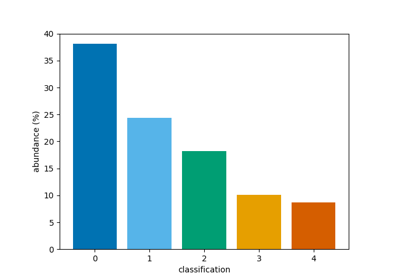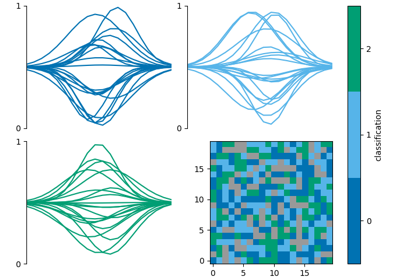# bar

mcalf.visualisation.bar(class_map=None, vmin=None, vmax=None, reduce=True, style='original', cmap=None, ax=None, data=None)[source]

Plot a bar chart of the classification abundances.

Parameters:
• class_map (numpy.ndarray[int], ndim=2 or 3) – Array of classifications. If the array is three-dimensional, it is assumed that the first dimension is time, and a time average classification will be plotted. The time average is the most common positive (valid) classification at each pixel.

• vmin (int, optional, default=None) – Minimum classification integer to plot. Must be greater or equal to zero. Defaults to min positive integer in class_map.

• vmax (int, optional, default=None) – Maximum classification integer to plot. Must be greater than zero. Defaults to max positive integer in class_map.

• reduce (bool, optional, default=True) – Whether to perform the time average described in class_map info.

• style (str, optional, default='original') – The named matplotlib colormap to extract a `ListedColormap` from. Colours are selected from vmin to vmax at equidistant values in the range [0, 1]. The `ListedColormap` produced will also show bad classifications and classifications out of range in grey. The default ‘original’ is a special case used since early versions of this code. It is a hardcoded list of 5 colours. When the number of classifications exceeds 5, `style='viridis'` will be used.

• cmap (str or matplotlib.colors.Colormap, optional, default=None) – Parameter to pass to matplotlib.axes.Axes.imshow. This parameter overrides any cmap requested via the style parameter.

• ax (matplotlib.axes.Axes, optional, default=None) – Axes into which the velocity map will be plotted. Defaults to the current axis of the current figure.

• data (dict, optional, default=None) – Dictionary of common classification plotting settings generated by `init_class_data()`. If present, all other parameters are ignored except and ax.

Returns:

b – The object returned by `matplotlib.axes.Axes.bar()` after plotting abundances.

Return type:

matplotlib.container.BarContainer

`mcalf.models.ModelBase.classify_spectra`

Classify spectra.

`mcalf.utils.smooth.average_classification`

Average a 3D array of classifications.

Notes

Visualisation assumes that all integers between vmin and vmax are valid classifications, even if they do not appear in class_map.

ExamplesPlot a bar chart of classifications

Plot a bar chart of classificationsCombine multiple classification plots

Combine multiple classification plots